Coplanar homework Help at TutorEye

## Top Questions

erature and pressure? Record your answer in scientific notation using 3 significant figures.
View More

## Coplanar

When the atoms present in a compound are lying in the same plane they are called coplanar. For example- Ethyne molecule.

## Coplanar Sample Questions

Question 1: What is coplanarity in chemistry?

a) The atoms of molecules are the same.                  b) The atoms of a molecule are single bonded.
c) The atoms lie in the same plane.                           d) All of the above.

Answer: Option c) All the atoms lie in the same plane.

Explanation: When things are coplanar it means it lies in the same plane. There is an x, y, and z plane. So, if atoms in molecule are coplanar, they would be in the same plane

Question 2: Atoms in which of the following molecules are coplanar?

a)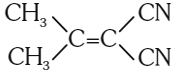b)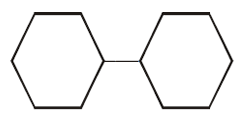c)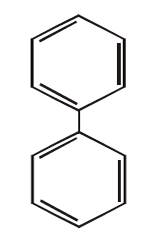d)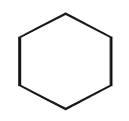Answer: Option c)Explanation: Biphenyl is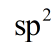hybridised. Molecules withHybridisation are planar in nature. therefore,is coplanar.

Question 3: In which geometry, all the atoms are coplanar?

a) Tetrahedral                        b) Trigonal bipyramidal
c) Pyramidal.                         d) Trigonal planar

Explanation: In trigonal planar geometry, all four atoms are present in the same plane and hence, they are coplanar. In the rest of the mentioned geometries, there are some atoms lying perpendicular to other atoms.

Question 4: Which of the following molecules has all the atoms coplanar?

a)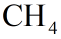b)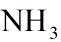c)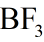d)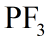Answer: Option c)Explanation:ishybridised and has 3 bonds and 0 lone pairs. The molecule has trigonal planar geometry with all the atoms lying in one plane and thus , all the atoms are coplanar.

Question 5: Atoms are coplanar in hybridization of -

a)b)c)d)Answer: Option b)Explanation:hybridized atoms are coplanar, as the geometry of such molecules is planar and atoms lie in the same plane and are coplanar.

Question 6: In which of the following geometry, all the atoms are not coplanar?

a) Tetrahedral                        b) Linear
c) Square planar                   d) Trigonal planar

Explanation: When the atoms present in a compound are lying in the same plane they are called coplanar. In tetrahedral geometry there are a total of five atoms but only three are coplanar and the other two are at angle with them.

Question 7: Which of the following statement is true-

a) All atoms are coplanar in pyramidal geometry                                                      b) Hybridizationhave all atoms coplanar.
c) All hydrogens and carbons are coplanar in methane.                                           d) Aromatic compounds are coplanar.

Answer: Option d)  Aromatic compounds are coplanar.

Explanation: The atoms involved in the ring are in the same plane and therefore the structure of aromatic compounds are coplanar.

Question 8: Which of the molecules is nonplanar?

a)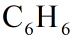b)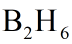c)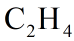d)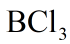Answer: Option b)Explanation: Diboraneshas non planar structure because two of the hydrogen are present above and below the boron and hydrogen bond.

Question 9: Which of the following statements is incorrect?

a) Coplanar atoms lie in one plane.                                                 b) Square pyramidal geometry has all the coplanar atoms.
c) Molecules with hybridisation has coplanar atoms.                      d) Carbon Dioxide is coplanar

Answer: Option b) Square pyramidal geometry has all the coplanar atoms.

Explanation: In square pyramidal geometry there are a total of 6 atoms present. Five of the atoms are present in one plane and the sixth one is perpendicular to them. Thus, not all the atoms are coplanar in square pyramidal geometry.

Question 10: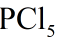is a planar molecule

a) True                        b) False

Explanation: Geometry ofis trigonal bipyramidal, P and the four Cl atoms lie in one plane and one of the Cl atom lie above the plane and one lies below the plane. Thereforeis nonplanar.

### What is coplanar?

When all the atoms present in a compound are lying in the same plane they are called coplanar. For example- Ethyne molecule.
The aromatic compounds have atoms in the ring lying on one plane and that’s why aromatic compounds are coplanar.

### What are coplanar points?

Coplanar points are two or more points which are lying in one plane. Coplanar molecules include atoms in the same plane. For example, In a Biphenyl ring, all the carbon and hydrogen atoms lie in the same plane.

### What is the difference between coplanar and collinear?

Collinear points or atoms are atoms lying in one line while coplanar atoms means atoms lying in one plane. A molecule can or can’t be both collinear and coplanar at same time.